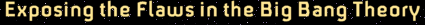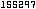# Ultimate Disproof of the Big Bang Comes from Its Bizarre Prediction that Photons Are Permanently Inscribed with H's Value at Time of Origin

### Abstract

A noted cosmologist has utilized big bang's expanding spacetime redshift expression, zexp = / e − 1, and the astronomical redshift expression, z = λ / λe − 1, to obtain an expression for the predicted present rate of photon wavelength change induced by expansion, here denoted by ( / dt)exp. When this expression is simplified in terms of the values of H, the present value of the Hubble constant, and He, its value at the time of emission of photons from distant galaxies, there results an expression showing the present rate of photon wavelength change depends on both the present value of the Hubble constant, as well as its value at the time of emission in distant galaxies, namely, ( / dt)exp = Hλ Heλe. This bizarre result requires two things: First, because of big bang's homogeneity assumption, all photons in the universe must be simultaneously undergoing the changes specified by this expression; this is nothing less than on-going, instantaneous action-at-a-distance all throughout the universe's thirty-billion-lightyear diameter. Second, the fact that it requires not only individual photons be initially imprinted with H's value, corresponding to their respective times of origin, but also that this imprint should determine the rate of present wavelength expansion is proof that the expansion hypothesis has always stood in contradiction to all of modern physics.

Parts 2 through 5 of this series have already shown several reasons why the F-L expansion postulate involved results and requirements, such as nonconservation of energy, that contradicted known physical laws. We now show its existence would have involved still another anomaly, thus far virtually overlooked.

In theory the expression, λ = ( / e)λe, says that all photons emitted at time te, with wavelength, λe, when the expansion factor was e, will now at time t, possess the same λ for the same . Thus, since the Friedmann-Lemaitre universe was presumed to be homogeneous and isotropic, then the rate of wavelength change, / dt, due to expansion at any specific point in the cosmos would have been instantaneously identical with the rate at any other point in the universe. And, since / dt was itself presumed to be changing, homogeneity would have required universal, instantaneous synchronization of this change in every photon throughout the universe's thirty-billion-light-year diameter. Since the CBR photon number density is about 410 cm−3, then the total number of photons throughout the cosmos in the CBR alone is more than 1085. Added to that number would of course be those in starlight and other forms of electromagnetic radiation. Thus, in some way that was never defined by modern physics, expansion's effects were required to instantly and synchronously induce specific wavelength changes to more than 1085 photons, irrespective of their location throughout the cosmos.

As we will now show, the foregoing conclusions are a direct consequence of Weinberg's attempt to determine if it was possible to detect a present-day change in a galaxy's redshift due to the effects of expansion . Thus we turn attention to Weinberg's derivation of expansion's presumed effect on the present rate of change of a photon's redshift, which is on page 451 of his book . If we let = (t) and e = (te) represent respectively the cosmic scale factors at some arbitrary time, t, at the present, and at some time of emission, te, from some distant galaxy, then the hypothesized expression for the expansion redshift is written as zexp = / e − 1

Differentiating this expression with respect to t, the present time coordinate, Weinberg first obtains

 dzexp / dt = [ℜe (dℜ / dt) − ℜ (dℜe / dte) (dte / dt)] / ℜe2, (1)

or

 dzexp / dt = [ℜeℜ⋅ − ℜℜ⋅e (dte / dt)] / ℜe2, (2)

and then utilizes the substitution dte / dt = e / from his equation 14.3.4 on page 416, to obtain

 dzexp / dt = ℜ⋅ / ℜe − ℜ⋅e / ℜe, (3)

which is his equation 14.6.23, as it appears on page 451. By definition the value of the Hubble constant at present is H = / , and its value at time te is presumed to have been He = e / e. If we first rewrite Eq. (3) as

 dzexp / dt = (ℜ⋅ / ℜ) (ℜ / ℜe) − ℜ⋅e / ℜe, (4)

and then utilize the foregoing values for the Hubble constant, we obtain

 dzexp / dt = H (ℜ / ℜe) − He. (5)

As noted in Part 2 of this series, for high z objects the expansion redshift, zexp = / e − 1, is assumed to be the main component of the observed redshift, zobs = λ / λe − 1. So in this case big-bang cosmology leads to the hypothesized expression,

 λ / λe = ℜ / ℜe. (6)

Since λe is always assumed a constant, then from zobs = λ / λe − 1 we find

 dzobs / dt = (dλ / dt) / λe. (7)

Substitution of Eqs. (6) and (7) into Eq. (5) yields

 dλ / dt = Hλ − Heλe. (8)

Before discussing the implications of Eq. (8), we pause to again define its parameters. The wavelength, λ, is presumed to be the redshifted analogue of λe, which represents the fixed, standard wavelength of any specific spectral line, as determined by an observer at the point of emission. All astronomical redshift calculations assume that λe is identical with the terrestrial laboratory standard for that spectral line. Thus, dλe / dt = dλe / dte = 0, which is why these terms are missing from Eqs. (7) and (8).

Equation (8) is extraordinary. It demands that, if the universe were governed by Friedmann-Lemaitre expansion, then every photon in the universe would now be experiencing a rate of wavelength change that is dependent on the present value of the Hubble constant, H, as well as its value at the time of emission, He, no matter when the emission occurred. Thus, any two presently adjacent photons having identical instantaneous values of λ , one born an hour ago, and the other in a distant galaxy, would exhibit different dλ / dt values because of intrinsic differences due to being imprinted at birth with different values of He. This means big-bang cosmology always required a photon's present physical characteristics be determined by a memory of the spacetime expansion conditions at the time of its origin, as well as instantaneous and synchronous action-at-a-distance throughout the cosmos, which also requires a universal time standard.

Part 2 of this series discussed how the effects of spacetime expansion were perceived by various cosmologists. It was noted that the contradictions were sufficient to show the theory must be flawed. Parts 3, 4, and 5 confirmed this, and the present discussion does even more. What is now reported is the discovery that if expansion ever existed, it would have required photons to forever retain an imprint of the Hubble constant at their respective times of emission. This result is so bizarre that it is sufficient in itself to falsify the expansion hypothesis, independent of any other considerations. Actually, however, this result in not surprising at all considering that it is critically hinged on the assumption of the validity of Eq. (6), which, as Part 5 showed, is the fatally flawed connecting link between the real world of astronomy and the imaginary world of spacetime expansion. In one sense we have discovered a second falsification of the expansion hypothesis. It is somewhat puzzling how this extraordinary feature remained undiscovered until now .

### References

 Steven Weinberg, Gravitation and Cosmology, (John Wiley& Sons, 1972) pp. 416, 451.

 Many thanks to Dave Gentry for useful discussions.The Ten
Censored
Papers

PDF
Version

Math
characters
not right?